Courses

# Heights and Distance - Important Formulas; Logical Reasoning LR Notes | EduRev

## Logical Reasoning (LR) and Data Interpretation (DI)

Created by: Bakliwal Institute

## LR : Heights and Distance - Important Formulas; Logical Reasoning LR Notes | EduRev

The document Heights and Distance - Important Formulas; Logical Reasoning LR Notes | EduRev is a part of the LR Course Logical Reasoning (LR) and Data Interpretation (DI).
All you need of LR at this link: LR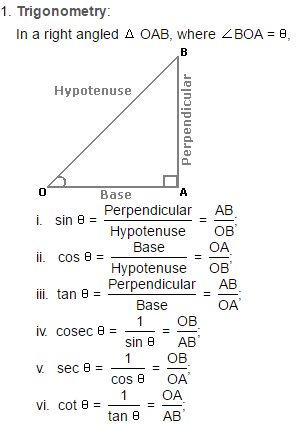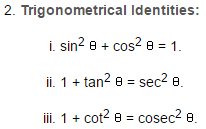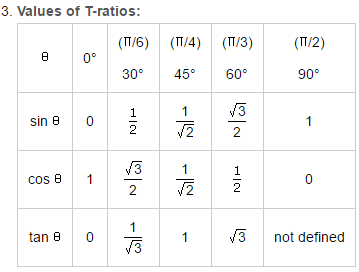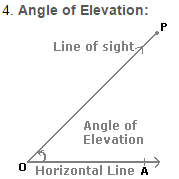Suppose a man from a point O looks up at an object P, placed above the level of his eye. Then, the angle which the line of sight makes with the horizontal through O, is called the angle of elevation of P as seen from O.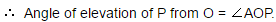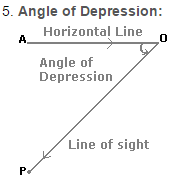Suppose a man from a point O looks down at an object P, placed below the level of his eye, then the angle which the line of sight makes with the horizontal through O, is called the angle of depression of P as seen from O.

## Logical Reasoning (LR) and Data Interpretation (DI)

44 videos|76 docs|119 tests

,

,

,

,

,

,

,

,

,

,

,

,

,

,

,

,

,

,

,

,

,

;